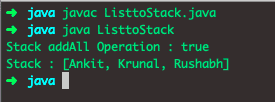# Stack Class in Java | Java Stack Class Example

Stack class in Java is a special kind of data structure which can be thought a linear structure represented by physical stack or pile. In a stack data structure, we can perform the insertion and deletion of items. In this data structure, we can add or remove an element from the top of the structure.

## Stack Class in Java Tutorial

Java Stack is the legacy Collection class. It extends the Vector class with five operations to support the LIFO(Last In First Out) operations. It is available in the Collection API since Java 1.0. As a Vector implements List, Stack class is also the List implementation class but does NOT support all the operations of Vector or List. As Stack supports LIFO, it is also known as LIFO Lists. Java is an Object-Oriented programming language as well all know.A pile of books/ pile of a dish is a real-life example of the Stack Data structure. We can easily understand from this above example is that if we want to remove elements from the stack, we must remove an item from its top. So, the element which we will insert at the last that item has to remove first. This algorithm is known as LIFO (Las-in-First-Out). There are two types of operations in Stack data structure, i.e., push (inserting element into the stack) and pop (removing items from the stack).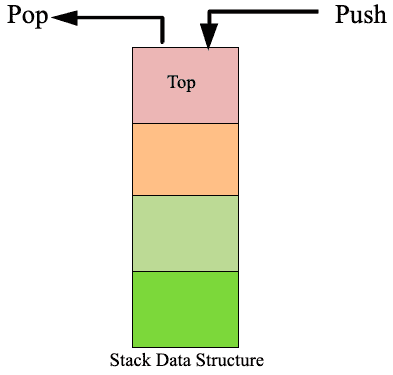Now let’s talk about the Stack class in Java. Collection framework takes cares of Stack class in Java. Stack class is a subclass of Vector, and this Vector class implements List, Collection, and Iterable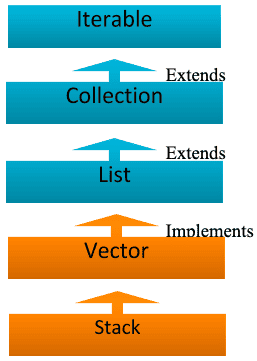## Stack Constructor

Stack class only supports one default constructor which is Stack();

It is used to create an empty stack.

## Java Stack Example

See the following necessary example code of Stack Data Structure in Java.

```// StackDemo.java

import java.util.Stack;

class StackDemo {
public static void main(String a[]){
Stack<Integer> stack = new Stack<>();
System.out.println("Empty stack : "  + stack);
System.out.println("Empty stack : "  + stack.isEmpty());

stack.push(100);
stack.push(102);
stack.push(103);
stack.push(104);
System.out.println("Stack elements are : "  + stack);
System.out.println("Stack: Pop Operation : "  + stack.pop());
System.out.println("Stack: After Pop Operation : "  + stack);
System.out.println("Stack : search() Operation : "  + stack.search(1002));
System.out.println("If Stack is empty : "  + stack.isEmpty());
}
}```

See the following output.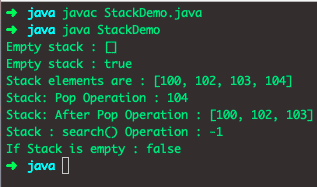In the above program, we have seen almost all the methods of Stack class in Java, but we will see each method in detail in the next portion of this article.

## Methods in Stack Class in Java

Here we’ll be going to discuss some critical methods in stack class which perform insertion, deletion, search operation in Stack class.

## Object push(Object element) in Stack

It is used to insert a specific element into the stack and returns the inserted element.

See the following code.

```// Push.java

//importing stack class
import java.util.Stack;
class Push
{
public static void main(String[] er)
{
//creating instance of stack class
Stack <Integer> st=new Stack<>();

//inserting elements into the stack
st.push(10);
st.push(20);
st.push(30);
st.push(40);
st.push(50);
st.push(60);

//dispalying elements in the stack
System.out.println("Elements in the stack are:"+st);
}
}```

The output is following.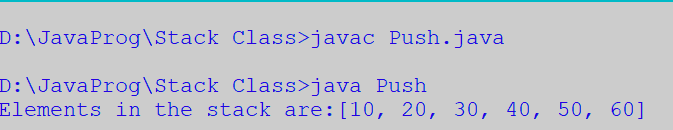## Object pop() in Stack

It is used to remove the top element from the stack and returns the removed element. See the program of a pop() method in Stack Class.

```// Pop.java

//importing stack class
import java.util.Stack;
class Pop
{
public static void main(String[] er)
{
//creating instance of stack class
Stack <Integer> st=new Stack<>();

//inserting elements into the stack
st.push(10);
st.push(20);
st.push(30);
st.push(40);
st.push(50);
st.push(60);

//dispalying elements in the stack
System.out.println("Elements in the stack are:"+st);

//poping top element from the stack
st.pop();
System.out.println("Elements in the stack after performing 1st pop() operation:"+st);
st.pop();
System.out.println("Elements in the stack after performing 2nd pop() operation:"+st);
}
}```

See the output.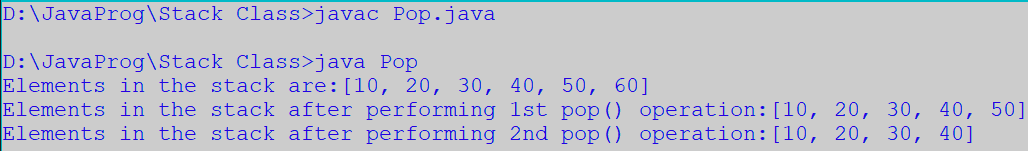## Object peek() method in Stack Class

The peek() method is used to return the first element of the stack, but it does not remove from the top.

See the example code of peek() method in Stack.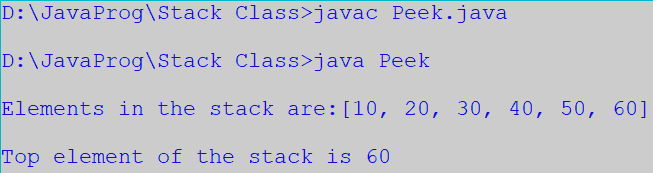## boolean empty() method in Stack

The boolean empty() method is used to test if the stack is empty or not. If the stack is empty, it returns true else false. See the example code of the empty() method in Stack class.

```// Empty.java

//importing stack class
import java.util.Stack;
class Empty
{
public static void main(String[] er)
{
//creating instance of stack class
Stack <Integer> st=new Stack<>();

//checking weather there is any element or not using empty() method

//inserting elements into the stack
st.push(10);
st.push(20);
st.push(30);
st.push(40);
st.push(50);
st.push(60);

//after adding elements, checking weather the stack is empty or not
//dispalying elements in the stack
System.out.println("\nElements in the stack are:"+st);

}
}```

See the following output.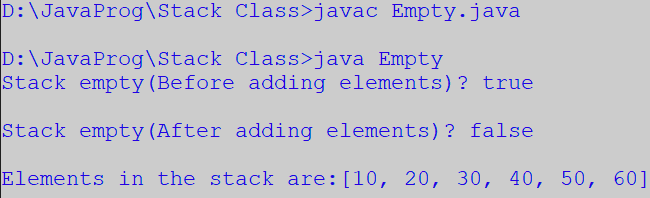## int search (Object element)

The search() method returns a position of the specific object.

See the following code example of search() method in Java Stack class.

```// Search.java

//importing stack class
import java.util.Stack;
class Search
{
public static void main(String[] er)
{
//creating instance of stack class
Stack <Integer> st=new Stack<>();

//inserting elements into the stack
st.push(10);
st.push(20);
st.push(30);
st.push(40);
st.push(50);
st.push(60);

//dispalying elements in the stack
System.out.println("\nElements in the stack are:"+st);

//checking the position of the specific element in the stack

System.out.println("\nPosition of the element '60' in the satck is "+st.search(60));

}
}```

See the following output.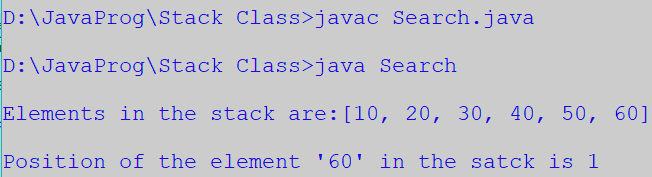### How Stack’s push() and pop() operations work Internally?

Stack’s push() and pop() are the most frequently used Stack operations. The push() operation is used to insert an item into the Stack at the top. The pop() operation is used to remove a top item from a Stack.

The stack data structure has one internal property: top which refers to the top element of that stack. If Stack is empty, the top refers to before the first element. Stack’s Push operation always inserts a new item at the top of the Stack.  Stack’s Pop operation always removes the element from the top of a Stack.

## Java Array to Stack Example

How to create the Stack object with a given Int array.

```// ArrayToStackExample.java

import java.util.Stack;

public class ArrayToStackExample {
public static void main(String a[]){
Integer[] intArr = { 100,102,103,104};
Stack<Integer> stack = new Stack<>();
for(Integer i : intArr){
stack.push(i);
}
System.out.println("Stack : "  + stack);
}
}```

See the output.## Java List to Stack Example

Let’s see How to create a Stack object with a given List of Strings.

```// ListtoStack.java

import java.util.ArrayList;
import java.util.List;
import java.util.Stack;

public class ListtoStack {
public static void main(String a[]){
Stack<String> stack = new Stack<>();
List<String> list = new ArrayList<>();